# Factor pairs

Here you will learn about factor pairs, including how to find factor pairs for a given number.

Students will first learn about factor pairs as a part of operations and algebraic thinking in 4th grade.

## What are factor pairs?

Factor pairs are two numbers that are multiplied to create a particular product.

Numbers can have more than one factor pair.

All numbers have the factor of 1, therefore the first factor pair will always be 1 \, \times the given number.

For example, 1 and 20, \; 2 and 10, and 4 and 5 are factor pairs for 20.

Factor pairArray (row \textbf{×} columns)Product
1 \times 20
20
2 \times 10
20
4 \times 5
20

Factor pairs have a commutative property, which means you can switch the order and the product is the same.

For example,

2 \times 10 = 20

10 \times 2 = 20

The factors of 20 are:

Factors are numbers that multiply together to find a product. They will divide into a whole number with no remainder and can sometimes be called divisors.

For example, on the multiplication chart below, you can see that 2 and 10, and 4 and 5 are factors of 20.

Factors can be prime or composite.

Composite numbers are numbers with more than two factors and prime numbers are numbers with exactly two factors, themselves and 1.

For smaller numbers, being familiar with your multiplication facts will help you find all factor pairs. For larger numbers, being familiar with divisibility rules will help you find all the factor pairs.

You can use factor pairs to help find common denominators, calculate areas, and will help you with algebraic expressions in middle school.

### What are factor pairs?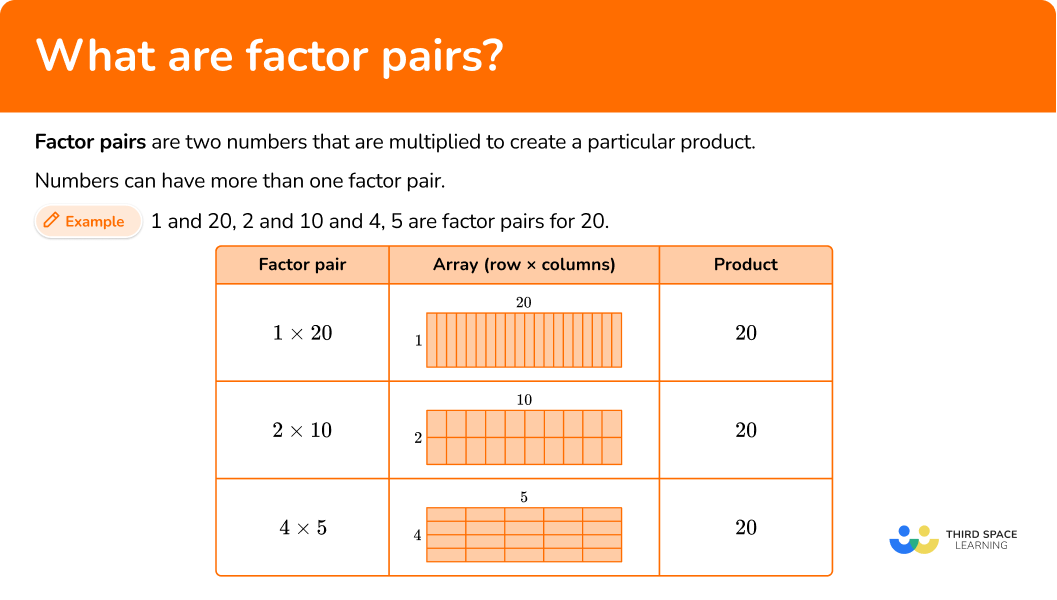## Common Core State Standards

How does this relate to 4th grade math?

• Grade 4: Operations and Algebraic Thinking (4.OA.B.4)
Find all factor pairs for a whole number in the range 1–100. Recognize that a whole number is a multiple of each of its factors. Determine whether a given whole number in the range 1–100 is a multiple of a given one-digit number. Determine whether a given whole number in the range 1–100 is prime or composite.

## How to find factor pairs of a number

In order to find factor pairs of a number, you need to:

1. State the pair \bf{1 \, \times } the number.
2. Find the next smallest factor of the number and calculate its factor pair.
3. Repeat until the next factor pair is the same as the previous pair.
4. Write out the list of factor pairs for the given number.

## Factor pairs examples

### Example 1: finding factor pairs (even number)

Find the factor pairs of 28.

1. State the pair \bf{1 \, \times } the number.

The factor pair is 1 \times 28.

2Find the next smallest factor of the number and calculate its factor pair.

28 is an even number, so 2 will be a factor of 28.

28 \div 2=14

So the next factor pair is,

2 \times 14

3Repeat until the next factor pair is the same as the previous pair.

28 is not divisible by 3 or 9 because the sum of its digits, 2+8=10, is not divisible by 3.

28 \div 4=7, so the next factor pair is 4 \times 7.

28 is not divisible by 5 because it does not end in a 0 or 5.

28 is not divisible by 6 because it is not divisible by 3.

The next factor to try is 7. As factors are communicative, 4 \times 7=7 \times 4, which is the same as the previous factor pair.

All of the factor pairs have been found.

4Write out the list of factor pairs for the given number.

The number 28 has three factor pairs:

\begin{aligned} & 1 \times 28 \\\\ & 2 \times 14 \\\\ & 4 \times 7 \end{aligned}

### Example 2: finding factor pairs (odd number)

Find the factor pairs of 35.

The factor pair is 1 \times 35.

35 is not an even number, so it is not divisible by 2 or 4.

35 is not divisible by 3 or 9 because the sum of its digits, 3 + 5 = 8, is not divisible by 3.

35 \div 5=7

So the next factor pair is,

5 \times 7

35 is not divisible by 6, because 35 is not divisible by 2 or 3.

The next factor to try is 7. As factors are communicative, 5 \times 7=7 \times 5, which is the same as the previous factor pair.

All of the factor pairs have been found.

The number 35 has two factor pairs:

\begin{aligned} & 1 \times 35 \\\\ & 5 \times 7 \end{aligned}

### Example 3: finding factor pairs (prime number)

Find the factor pairs of 59.

The factor pair is 1 \times 59.

59 is not divisible by 2 or 4 because the last digit isn’t even.

59 is not divisible by 3 or 9 because the sum of the digits, 5+9=14, is not divisible by 3.

59 is not divisible by 5 because the last digit is not a 0 or 5.

59 is not divisible by 6 because the number is not divisible by 2 or 3.

59 is not divisible by 7 or 8 because 59 \div 7 and 59 \div 8 have remainders, and are not divided evenly.

59 does not have any other factors besides 1 or itself. 59 is a prime number.

The only factors of 59 are 1 and itself.

The number 59 only has one factor pair:

1 \times 59

### Example 4: finding factor pairs with factors greater than 12

Find the factor pairs of 64.

The factor pair is 1 \times 64.

64 ends in a 4, an even number, so 2 will be a factor of 64.

64 \div 2=32

So the next factor pair is,

2 \times 32

64 is not divisible by 3 or 9 because the sum of the digits, 6 + 4 = 10, is not divisible by 3.

64 is divisible by 4, because 64 \div 4=16

So the next factor pair is, 4 \times 16.

64 is not divisible by 5 because the last digit is not a 0 or 5.

64 is not divisible by 6 because the number is not divisible by 2 or 3.

64 is not divisible by 7 because 64 \div 7 has a remainder, and is not divided evenly.

64 is divisible by 8, because 64 \div 8=8

So the next factor pair is, 8 \times 8.

The factors began repeating each other, therefore, all factor pairs have been found.

The number 64 has 4 factor pairs:

\begin{aligned} & 1 \times 64 \\\\ & 2 \times 32 \\\\ & 4 \times 16 \\\\ & 8 \times 8 \end{aligned}

### Example 5: finding factor pairs with factors greater than 12

Find the factor pairs of 66.

The factor pair is 1 \times 66.

66 ends in a 6, an even number, so 2 will be a factor of 66.

66 \div 2=33

The next factor pair is 2 \times 33.

66 is divisible by 3, because the sum of its digits, 6+6=12, is divisible by 3.

66 \div 3=22

The next factor pair will be 3 \times 22.

66 is not divisible by 4, because 66 \div 4 has a remainder, and is not divided evenly.

66 is not divisible by 5, because the last digit is not a 0 or 5.

66 is divisible by 6, because it is divisible by both 2 and 3.

66 \div 6=11

The next factor pair will be 6 \times 11.

66 is not divisible by 7 or 8, because 66 \div 7 and 66 \div 8 have remainders, and are not divided evenly.

66 is not divisible by 9, because the sum of its digits, 6+6=12, is not divisible by 9.

All factor pairs have been found.

The number 66 has 4 factor pairs:

\begin{aligned} & 1 \times 66 \\\\ & 2 \times 33 \\\\ & 3 \times 22 \\\\ & 6 \times 11 \end{aligned}

### Example 6: finding factor pairs (three digit number)

Find the factor pairs of 121.

The factor pair is 1 \times 121.

121 is not divisible by 2 or 4 because the last digit isn’t even.

121 is not divisible by 3 or 9 because the sum of the digits, 1 + 2 +1 = 4, is not divisible by 3.

121 is not divisible by 5 because the last digit is not a 0 or 5.

121 is not divisible by 6 because the number is not divisible by 2 or 3.

121 is not divisible by 7 or 8 because 89 \div 7 and 89 \div 8 have remainders, and are not divided evenly.

121 is not divisible by 10 because it does not end in a zero.

121 is divisible by 11, because 121 \div 11 = 11.

All factor pairs have been found.

The number 121 has two factor pairs:

\begin{aligned} & 1 \times 121 \\\\ & 11 \times 11 \end{aligned}

### Teaching tips for factor pairs

• Using some type of visual model is a great way to introduce factor pairs. Students should be familiar with arrays by this grade level and can help students organize the positive factors.

• While worksheets and assigning as math homework are an easy way to provide students practice with factor pairs, when students are first working with them, providing hands-on practice, like manipulatives, will help students cement the learning.

### Easy mistakes to make

• Confusing factors and multiples
Factors are two numbers multiplied together to get a product, like 6 \times 7 = 42.
Multiples are the products of factors, like the multiples of 4 are 4, 8, 12, 16.

• Remember \bf{1} and the number itself are factors
All numbers have a factor of 1, and all numbers are a factor of themselves. For example, the factors of 4 are: 1, 2, and 4.

### Practice factor pairs questions

1. Which of the following is a factor pair of 75?

5 \times 152 \times 384 \times 183 \times 24You can use divisibility rules to determine which of the answer choices is a factor pair of 75.

• 75 is not divisible by 2 because the last digit is not even or a 0.
• 75 is not divisible by 3 because the sum of the digits, 7+5=13, is not divisible by 3.
• 75 is not divisible by 4 because 75 \div 4, gives you a remainder.
• 75 is divisible by 5 because the last digit is 5.

The factor pairs for 75 are: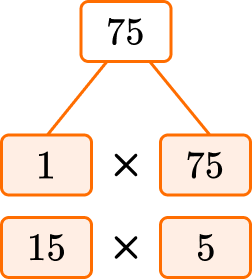2. Which of the following is NOT a factor pair of 56?

2 \times 287 \times 83 \times 154 \times 14You can use divisibility rules to determine which of the answer choices is NOT a factor pair of 56.

• 56 is divisible by 2 because 6 is an even number.
• 56 is not divisible by 3 because the sum of the digits, 5+6=11, is not divisible by 3.
• 56 is not divisible by 4 because 56 \div 4, gives you a remainder.
• 56 is not divisible by 7 because 56 \div 7, gives you a remainder.

The factor pairs for 56 are: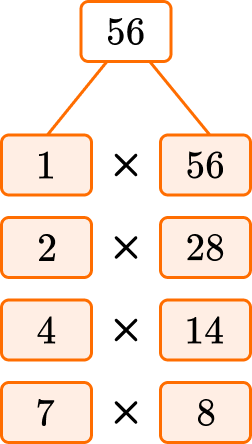3. Which of the following is a factor pair of 49?

5 \times 97 \times 72 \times 243 \times 16You can use divisibility rules to determine which of the answer choices is a factor pair of 49.

• 49 is not divisible by 2 because 9 is not an even number or zero.
• 49 is not divisible by 3 because the sum of the digits, 4+9=13, is not divisible by 3.
• 49 is not divisible by 5 because the last digit is not 0 or 5.
• 49 is not divisible by 7 because 49 \div 7, gives you a remainder.

The factor pairs for 49 are: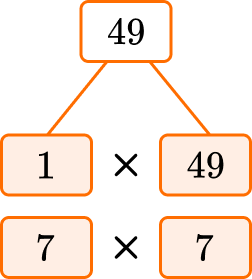4. Which of the following is NOT a factor pair of 88?

2 \times 444 \times 228 \times 113 \times 29You can use divisibility rules to determine which of the answer choices is NOT a factor pair of 88.

• 88 is divisible by 2 because 8 is an even number.
• 88 is not divisible by 3 because the sum of the digits, 8+8=16, is not divisible by 3.
• 88 is divisible by 4 because 88 \div 4 = 22.
• 88 is divisible by 8 because 88 \div 8 = 11.

The factor pairs for 88 are: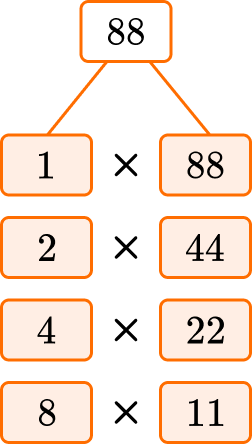5. Which of the following is a factor pair of 95?

9 \times 105 \times 194 \times 236 \times 15You can use divisibility rules to determine which of the answer choices is a factor pair of 95.

• 95 is not divisible by 4 because 95 \div 4 gives you a remainder.
• 95 is divisible by 5 because it ends with 5.
• 95 is not divisible by 6 because it is not divisible by 2 or 3.
• 95 is not divisible by 9 because the sum of the digits, 9+5=14, is not divisible by 9.

The factor pairs for 95 are: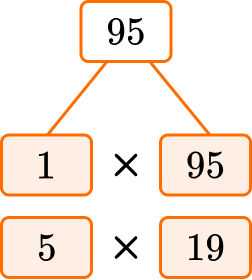6. Which of the following is NOT a factor pair for 60?

2 \times 304 \times 155 \times 146 \times 10You can use divisibility rules to determine which of the answer choices is NOT a factor pair of 60.

• 60 is divisible by 2 because the last digit is 0.
• 60 is not divisible by 4 because 60 \div 4 gives you a remainder.
• 60 is divisible by 5 because it ends with 0.
• 60 is divisible by 6 because it is divisible by 2 and 3.

The factor pairs for 60 are: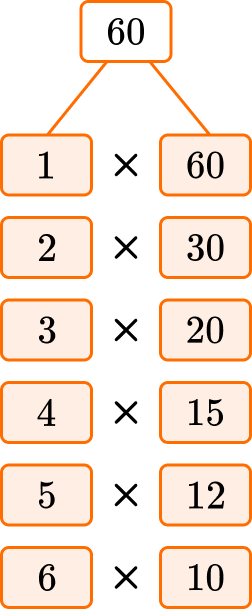## Factor pairs FAQs

Can factor pairs only contain positive numbers?

No, factors or negative numbers can be negative numbers as well. However, in elementary school students will only work with positive numbers.

How else will I use factor pairs?

Factor pairs will be used to help find the common denominators, including the greatest common factor, or GCF.

## Still stuck?

At Third Space Learning, we specialize in helping teachers and school leaders to provide personalized math support for more of their students through high-quality, online one-on-one math tutoring delivered by subject experts.

Each week, our tutors support thousands of students who are at risk of not meeting their grade-level expectations, and help accelerate their progress and boost their confidence.